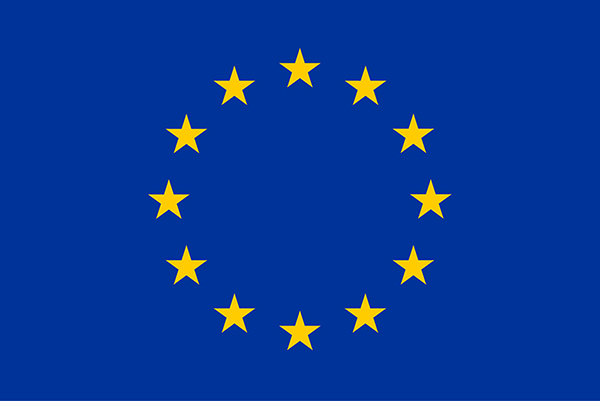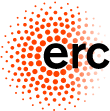# Homotopy Probability Theory

## Workshop in Saarbrücken, 04 September - 08 September, 2017Funded by the ERC Advanced Grant Non-Commutative Distributions in Free Probability

Organised by Roland Friedrich and Carlos Vargas Obieta.

Participants
Aim of the workshop
Accommodation
Schedule
Talks & Abstracts

### Participants

• Drummond-Cole, Gabriel   (IBS Center for Geometry and Physics)
• Ebrahimi-Fard, Kurusch   (Norwegian University of Science and Technology NTNU)
• Friedrich, Roland   (Saarland Universität)
• Frosini, Patrizio   (Università di Bologna)
• Gwilliam, Owen   (MPI für Mathematik Bonn)
• Koestler, Claus   (University College Cork)
• Lehner, Franz   (TU Graz)
• Muraki, Naofumi,   (Iwate Prefectural University)
• Park, Jae-Suk   (IBS Center for Geometry and Physics, POSTECH)
• Patras, Frédéric    (Université de Nice Sophia-Antipolis, UMR CNRS-UNS)
• Rieser, Antonio    (CONACYT-CIMAT)
• Speicher, Roland    (Saarland Universität)
• Vargas-Obieta, Carlos    (CONACYT-CIMAT)
• Voiculescu, Dan-Virgil   (University of California, Berkeley)
• Tarrago, Pierre   (CONACYT-CIMAT)
• Thorbjørnsen, Steen   (Aarhus University)

### Aim of the workshop

Homotopy Probability Theory is a recent development, which enriches the notion of an algebraic probability space (as used in non-commutative and free probability theory) with ideas from algebraic homotopy theory. It was originally initiated by Jae-Suk Park, motivated by quantum field theory, and also based on a surprising coincidence between morphisms of homotopy operadic algebras and cumulant functions (classical, free, boolean) in probability theory.
Topological Data Analysis, and in particular persistent homology, comprises a set of methods from algebraic topology in order to determine topological features of a data space at various scales. The proper features, which are not due to noise or other parameters, should be present over larger scales and hence characterise the underlying data more faithfully.
The aim of the workshop is to bring together people from different fields in mathematics, such as algebraic probability theory, mathematical physics, algebra and stochastic topology (topological data analysis) and to discuss further developments and applications of the theory. In particular, we expect to find rich interactions between the domains mentions above which should lead to new insights into the respective fields itself.
We intend to organise our meeting more as a workshop rather than a conference, with time for public and private discussions.

### Accommodation

All participants are accommodated in Hotel Madeleine downtown Saarbruecken. Fur further information how to get to the hotel and how to get to the math department, see our webpage with information for visitors.

-->

### Schedule

(first version, up to changes)
Each talk consists of 50 minutes plus 10 minutes discussion.
All lectures take place in lecture hall IV, building E2 4. This lecture hall is equipped with a projector and blackboards.

The coffee breaks and the reception are on ground floor. The seminar rooms Seminarraum 10 and Zeichensaal can be used for private discussions during the conference.

A pdf version of the program (will be distributed in printed form also during the reception).

Monday, 04 September
 10:00 11:00 12:30 2:00 pm 3:00 pm 3:30 pm Reception in the basement of building E2 4 Gwilliam Lunch Vargas-Obieta Coffee Koestler
Tuesday, 05 September
 10:00 11:00 11:30 12:30 2:00 pm 3:00 pm 3:30 pm Park Coffee Frosini Lunch Patras Coffee Lehner
Wednesday, 06 September
 10:00 11:00 11:30 12:30 2:00 pm 7:00 pm Frosini Coffee Park Lunch Free afternoon or social gathering Dinner
Thursday, 07 September
 10:00 11:00 11:30 12:30 2:00 pm 3:00 pm 3:30 pm Drummond-Cole Coffee Rieser Lunch Ebrahimi-Fard Coffee Friedrich
Friday, 08 September
 10:00 11:00 11:30 12:30 Ebrahimi-Fard Coffee Rieser Lunch
The workshop will end on Friday, September 08, 2017 at 2:00 pm

### Talks & Abstracts

• Drummond-Cole, Gabriel
A homotopy-algebraic point of view on free probability  Homotopy probability theory describes moment-cumulant inversion formulas in terms of morphisms of homotopy algebras. I will describe a type of homotopy algebra that enacts this framework in the case of free cumulants with commutative target.

• Ebrahimi-Fard, Kurusch
Probability and shuffle products II: moment-cumulant relations and shuffle-exponentials  In this talk we consider monotone, free, and boolean moment-cumulant relations from the shuffle algebra viewpoint. Cumulants are described as infinitesimal characters over a particular combinatorial Hopf algebra, which is neither commutative nor cocommutative. As a result the moment-cumulant relations can be encoded in terms of shuffle and half-shuffle exponentials. Those shuffle exponentials and the corresponding logarithms permit to express monotone, free, and boolean cumulants in terms of each other using the pre-Lie Magnus expansion.

• Ebrahimi-Fard, Kurusch
Probability and shuffle products III: additive convolutions  Commutative shuffle products are intimately related to universal formulas in group theory as well as in the theory of free Lie algebras, such as, for instance, the Baker-Campbell-Hausdorff formula. Non-commutative shuffle products happen to have similar properties, however, with respect to pre-Lie algebras. In the second talk we saw that in the non-commutative framework three exponential maps and the corresponding logarithms are naturally defined. We explore these developments in the context of non-commutative probability theory by revisiting additive convolution in monotone, free and boolean probability and related aspects.

• Friedrich, Roland
Types, Structures and (Free) Harmonic Analysis  We shall discuss, from the perspective of universal algebra and operads, the analytic and algebraic structures which govern both the classical and free convolution of infinitely divisible probability measures. Further, we shall have a new look at the nature of moments and cumulants from this point of view.

• Frosini, Patrizio
An approach to topological data analysis via persistent topology and invariant operators.  In this talk we illustrate a metric approach to TDA that is based on persistent topology and a mathematical formalization of the concept of observer, seen as a collection of suitable operators acting on a metric space of functions. These functions represent the set of data that are accessible to the observer, while the operators describe the way the observer elaborates the data and enclose the invariance that he/she associates with them. We expose this model and show how it can benefit from the use of group-invariant persistent topology.

• Frosini, Patrizio
Some advances in the application of group-invariant persistent homology to topological data analysis.  Persistent homology has proven itself efficient in the topological and qualitative comparison of filtered topological spaces, when invariance with respect to every homeomorphism is required. However, we can make the following two observations about the use of persistent homology for application purposes. On the one hand, more restricted kinds of invariance are sometimes preferable (e.g., in shape comparison). On the other hand, in several practical situations filtering functions are not just auxiliary technical tools that can be exploited to study a given topological space, but instead the main aim of our analysis. In fact, most of the data is usually produced by measurements, whose results are quite often functions defined on a topological space. As a consequence, in many applications the dataset of interest is seen as a collection Phi of real-valued functions defined on a given topological space X, instead of a family of topological spaces. In these cases observers can be seen as collections of suitable operators on Phi. Starting from these remarks, we describe a way to combine persistent homology with the use of G-invariant non-expansive operators defined on Phi, where G is a group of self-homeomorphisms of X. This approach gives us a method to study Phi in a way that is invariant with respect to G. In our talk we will describe this general framework and present the use of group-invariant persistent homology for topological data analysis, focusing on some recent developments in the study of our model.

• Gwilliam, Owen
Formal matrix integrals via a quantization of the Loday-Quillen-Tsygan theorem  Starting with 't Hooft, physicists have used a ribbon graph expansion to understand certain integrals over spaces of N-by-N matrices in the large N limit. This expansion can be deduced from the Feynman diagram expansion, which relies on the nice structure of moments of a Gaussian measure. We provide a homological perspective on this situation: the Batalin-Vilkovisky formalism (which we will outline) provides a homological approach to computing moments, and the Loday-Quillen-Tsygan theorem (which we will explain) gives a method for identifying the large N limit. Our talk will focus on finite-dimensional integrals, but we comment on how our methods extend to gauge theories and connect with string field theory.

• Koestler, Claus
Algebraic central limit theorems from distributional symmetries  Distributional symmetries for non-commutative random variables are strong enough to provide algebraic central limit theorems. I will illustrate this in the framework of the infinite symmetric group when equipped with a certain length function. Somewhat unexpectedly, the resulting central limit law turns out to be directly related to the distribution of a GUE random matrix. My talk is based on joint work with Alexandru Nica.

• Lehner, Franz
Spreadability, Cumulants and Hausdorff Series  Spreadability systems generalize exchangeability systems in the same way quasisymmetric functions generalize symmetric functions. We investigate "cumulants" in this setting, in particular including monotone cumulants. As another application, eulerian Lie projectors can be interpreted as cumulants and yield yet another derivation of the Baker-Campbell-Hausdorff series. This is joint work with T. Hasebe.

• Park, Jae-Suk
Homotopy Theory of Probability Spaces  The notion of a homotopy probability space is an enrichment of the notion of an algebraic probability space with ideas from algebraic homotopy theory. This enrichment uses a characterization of the laws of random variables in a probability space in terms of symmetries of the expectation. The laws of random variables are reinterpreted as invariants of the homotopy types of infinity morphisms between certain homotopy algebras. The relevant category of homotopy algebras is determined by the appropriate notion of independence for the underlying probability theory. This talk will be focused on the commutative case, where the laws of random variables are also described in terms of certain affinely flat structures on the formal moduli space of a naturally defined family attached to the given algebraic probability space. Non-commutative theory with the free independence will be discussed in the talk of Gabriel Drummond-Cole. Time permitting, I will also sketch the similar but more sophisticated story on algebraic quantum field theory.

• Patras, Frédéric
Probability and shuffle products I: shuffle bialgebras  This is the first of three talks on the shuffle algebra approach to moment-cumulant relations in probability. We will introduce the classical, i.e., commutative shuffle algebra together with examples. Particular attention is given to the use of shuffle algebra in classical probability. We then introduce the notion of shuffle bialgebra and consider the question of how to approach non-commutative problems in, e.g., topology, matrices of shuffle algebras, and free probability.

• Rieser, Antonio
An introduction to algebraic topology, stochastic topology, and topological data analysis  In these talks, we will outline the current state of the two complementary fields of stochastic topology and topological data analysis, emphasizing areas where a non-commutative point of view is either already used in a rudimentary way, or very natural but as yet unexplored. Time permitting, we will also give a brief introduction to several (not necessarily topological) data analysis problems which we believe would benefit from a non-commutative approach.

• Vargas Obieta, Carlos
Non-commutative distributions for simplicial complexes  Graphs are natural candidates for scalar-valued non-commutative random variables via their adjacency matrices. The simplest examples of non-commutative random variables are exactly through graph products. We discuss the generalization of the notion of a non-commutative distribution for higher dimensional simplicial complexes. A nice, simple answer requires to consider the complexes as operator-valued non-commutative random variables. Important objects in algebraic topology, such as the Betti numbers, are carefully encoded in their operator-valued distributions.

 Updated: 07 September 2017   Friedrich Roland; friedrich[at]math.uni-sb.de Impressum